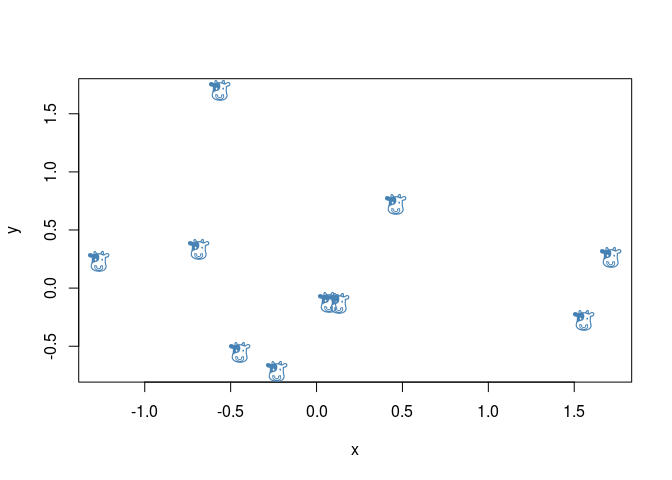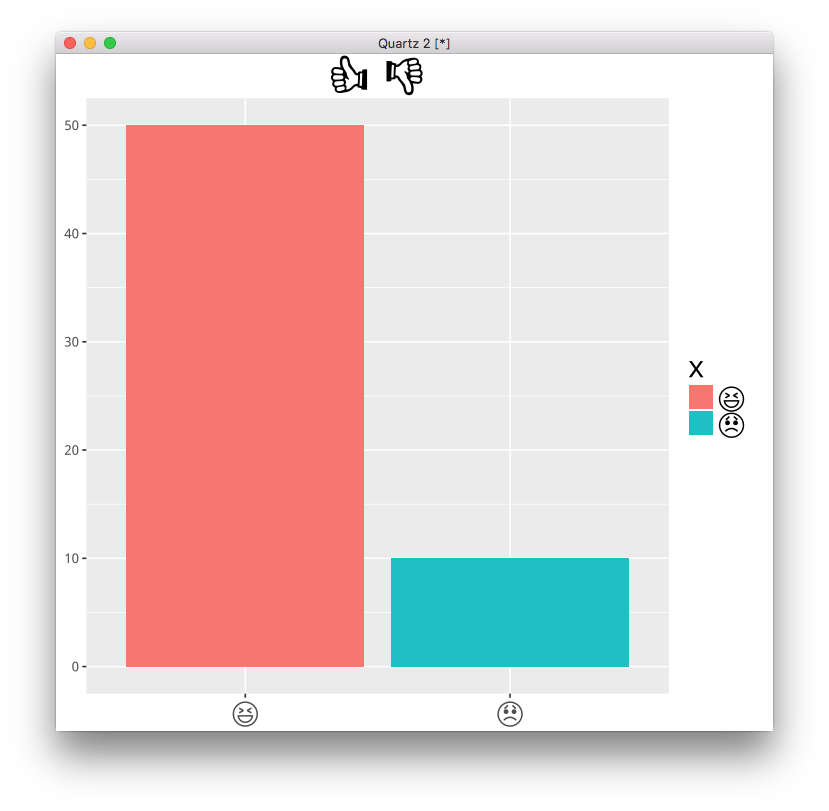`emojifont` is an implementation of using emoji font in both base and 'ggplot2' graphics. `emojifont` is released on CRAN and the source code is hosted on GitHub.

## Author

Guangchuang Yu, School of Public Health, The University of Hong Kong.

## Installation

To install `emojifont`, start R and enter:

``````install.packages("emojifont")
``````

## Examples

#### base plot

``````require(remoji)
set.seed(123)
x <- rnorm(10)
set.seed(321)
y <- rnorm(10)
plot(x, y, cex=0)
text(x, y, labels=emoji('cow'), cex=1.5, col='steelblue', family='OpenSansEmoji')
``````#### ggplot2

``````dd=data.frame(x=emoji(c("satisfied", "disapointed")), y=c(50, 10))
emoji_text=element_text(family="OpenSansEmoji", size=20)
ggplot(dd, aes(x, y)) + geom_bar(stat='identity', aes(fill=x)) +
ggtitle(paste(emoji(c("+1", "-1")), collapse=" "))+
theme(axis.text.x = emoji_text, legend.text=emoji_text, title=emoji_text) +
xlab(NULL)+ylab(NULL)
``````#### ggtree

``````require(ggtree)
require(colorspace)

tree_text=paste0(
"(","(","(",
"(",
"(",
emoji("cow"), ",",
"(",
emoji("whale"),",",
emoji("dolphin"),
")",
"),",
"(",
emoji('pig2'),",",
emoji('boar'),
")",
"),",
emoji("camel"),
"),", emoji("fish"), "),",
emoji("seedling"), ");")

ggtree(read.tree(text=tree_text)) + xlim(NA, 7) +
geom_tiplab(family="OpenSansEmoji", size=10,
color=rainbow_hcl(8))
``````comments powered by Disqus